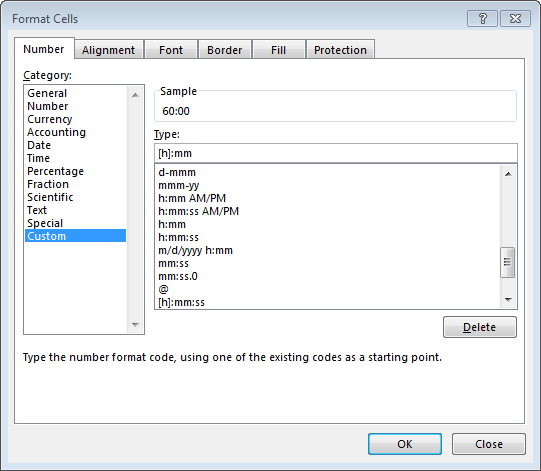# Clocking In

Have you ever tried to do some type of calculation with a time value in Excel, only to receive a strange result? Two examples are adding up hours on a time sheet and multiplying the number of hours worked by a pay rate. If you’ve tried something like this and encountered an unexpected result, the solution is fairly simple.

Dates and times are stored as decimal values, where the value to the left of the decimal represents the date and the value to the right represents the time. So, when you enter a date such as Jan. 1, 2017, Excel stores the value 42736. The date part is fairly easy to visualize, because the number 1 represents 1/1/1900, and Excel just adds 1 for each day.

The time part is more interesting. Since there are 24 hours in a day, Excel converts the number of hours into a decimal value by dividing by 24. For example, 6 a.m. is six hours, and six divided by 24 is 0.25. Noon is 0.5 and 6 p.m. is 0.75.

We can apply a variety of formats to display the stored value as desired. Since a cell’s default format is “General,” if you enter the value 42736 into a cell, you see that value. But, if you format the cell with one of the many “Date” formats available, then you see the expected date, such as 1/1/17 or January 1, 2017. When you enter a date that Excel recognizes, such as “1/1/17” into a cell, Excel stores the underlying date serial number.

The same applies to times. If you enter the value 0.25 into a cell that has “General” formatting, then you see the value 0.25. But, if you apply a time format, then you see the displayed time value, such as 6 a.m.

So far, so good? We’re just about done with the backstory, and then we’ll see how all of this fits together. We know that we can apply a date or time format to display the
desired date or time. But what happens if the stored value includes both a date and time?
For example, 42736.25.

It depends on the formatting. We could apply a date/time format to display both parts. If we apply a date format, Excel displays the date (value to the left of the decimal) but not the time (value to the right of the decimal).

Likewise, if we apply a time format, Excel displays the time, but not the date. When a time format is applied, the time of day is displayed. Remember this, because we’ll come back to it momentarily.

Let’s say we have a time sheet in Excel, it’s in the middle of busy season and we’re working crazy, 12-hour days. We enter 12:00 hours into 5 cells for Monday-Friday. When we write a formula to compute the total hours worked, we get the unexpected result 12:00. Let’s think about it for a moment.

The displayed value in each cell is 12:00, but the underlying stored value is 0.5. Add five days of 0.5, we get 2.5. When we apply a time format to a cell, the value to the right of the decimal is displayed, but not the value to the left. So, the formula displays 12:00.

So, what to do? The solution is to use the proper formatting. When we apply a time format, we are telling Excel to display a time of day. What we need is the duration, or elapsed time. When we say we started work at 8 a.m., that is a time of day. But, when we say we worked 12 hours, that is a duration. So, we simply need to apply a duration format to the cell, rather than a time format.

To do so, select the cell, then open the Format Cells dialog. From the Category list, select Custom. Then, enter the desired formatting code into the Type field. If you want to display a time of day, enter h:mm, for hours and minutes. To include seconds, the format code would be h:mm:ss. To convert these time formats into duration formats, we just use square brackets. For example, [h]:mm, as shown in Figure 1, would show the elapsed hours.Figure 1

When we enter a format code of [h]:mm and apply the format to our total cell, the displayed value is now 60:00 hours. And, five days at 12 hours per day is indeed 60 hours! So, when a duration format is applied, the elapsed time is displayed.

Now that we have 60:00 hours displayed in the cell, we need to compute the total pay. We do so by multiplying the hours worked by the \$10 hourly pay rate. When we write a formula that multiplies the total hours of 60 by \$10, and format it as a currency, the result is \$25. But, we expect the total to be \$600. We can explain this based on our discussion above.

We know the displayed elapsed time of 60:00 corresponds to a stored value of 2.5. Generally, Excel formulas operate on the stored value, not the displayed value. So, 2.5 times \$10 is \$25. Are we stuck? Nope. Recall that Excel divides the number of hours by 24 to compute the time value. We just need to reverse the math, and multiply by 24. Instead of 2.5 times \$10, our formula would be (2.5*24)*10, which produces \$600.

Since Excel handles dates and times this way, we have flexibility when displaying and working with dates and times. And remember, Excel rules!

Posted in### Jeff Lenning

I love sharing the things I've learned about Excel, and I built Excel University to help me do that. My motto is: Learn Excel. Work Faster.